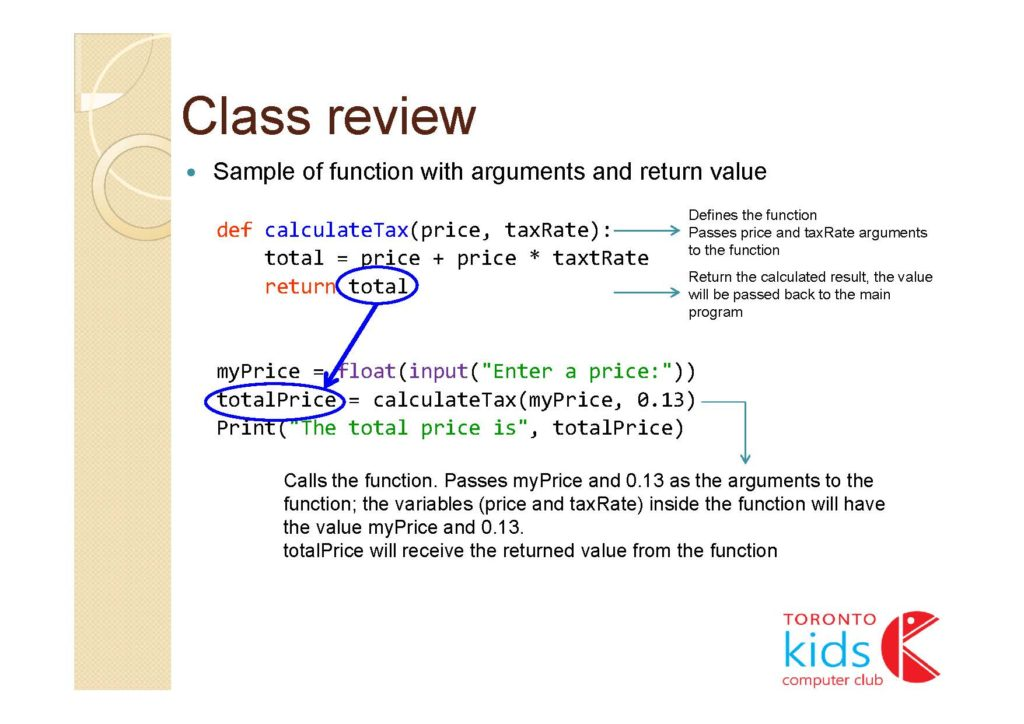TORONTO KIDS COMPUTER CLUB | Markham Friday 19:30 Python Homework 20.04.10.
17351

# Markham Friday 19:30 Python Homework 20.04.10.

## 17 Apr Markham Friday 19:30 Python Homework 20.04.10.

Question:

Write a function luckySum(a, b, c) to calculate sum of given 3 int values, a b c. The function will have a return value of their sum. However, if one of the values is 13 then it does not count towards the sum and values to its right do not count. So for example, if b is 13, then both b and c do not count.

Sample Input 1:

a: 1
b: 2
c: 3

Sample Output 1
The lucky sum is 6

Sample Input 2:

a: 1
b: 2
c: 13

Sample Output 2:
The lucky sum is 3

(Because c is 13, c does not count towards the sum)

Sample Input 3:

a: 1
b: 13
c: 3

Sample Output 3
The lucky sum is 1

(Because b is 13, both b and c does not count towards the sum)

Class review: